## Setting the quiz function

The Quiz function in Moodle is a tool for controlling the acquired knowledge of students.

Well-designed quizzes can be created easily from a pool (or bank) of questions. They are effective and make good use of tutor time, mainly if the computer is used to score everything. By setting advanced options we can allow students to retake quizzes for practice or optimize quizzes for assessment.

To create a quiz select Quiz from Adding an activity drop-down menu. Give the quiz a descriptive name and write an introduction including any special instructions for taking the quiz and for specific scoring rules.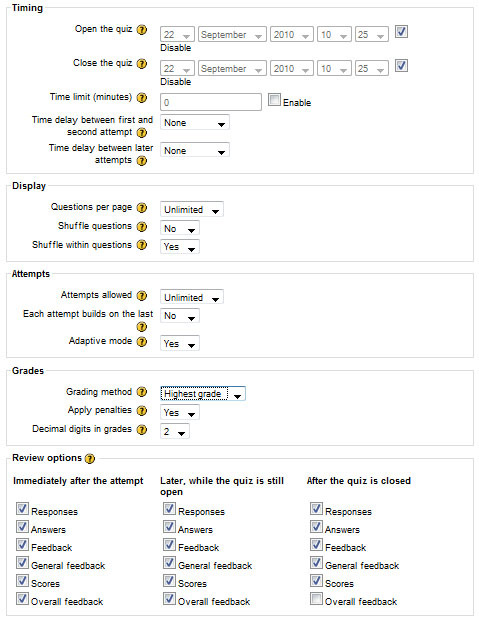There are a lot of options for displaying a quiz, as well as for its functions. Let's see them.

 Open the quiz / close the quiz The time period when the quiz is accessible for student attempts. Opening and closing time can be omitted. Time limit Students have to complete the quiz within that time. (different every time). In setting this time limit we limit the time students have to search for answers. Shuffle questions The order of questions in the quiz will be randomly shuffled, which also makes it harder for students to copy from each other. Shuffle within questions The order of answers within each question will be randomly shuffled for the reasons above. Attempts allowed The number of attempts a student can make. Each attempt builds on the last If multiple attempts are allowed then each new quiz contains the results of the previous attempt. Grading method The method to calculate the final grade. Students review options Immediately after finishing and grading the test selected options from the first editing screen will be displayed. It is advisable to turn this off if multiple attempts are allowed.

After settings click the Save changes button.

## Creating questions for a Quiz

Once we have saved our changes, we'll see the second editing screen where we can add questions to our quiz. In Moodle we can also use questions created for other courses. Select them from the Category drop-down menu.

Questions should ideally be organized into categories. The relation between these questions and categories are similar to files and folders on our computer. Categories can be created in a hierarchy, again like in a directory.

To use categories click on Categories tab.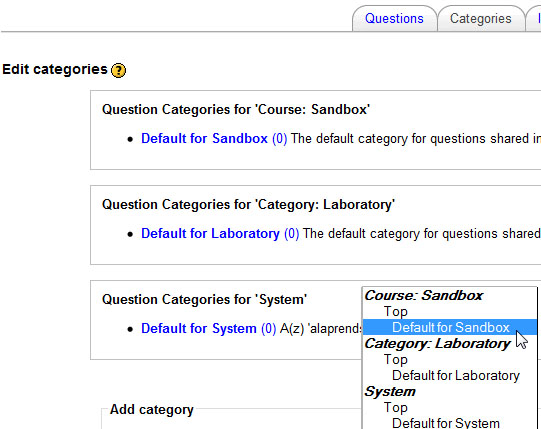Type the name of the new category. A description in the Category info area is useful, but not compulsory.

In the Parent drop-down menu we choose which category to place our new category in.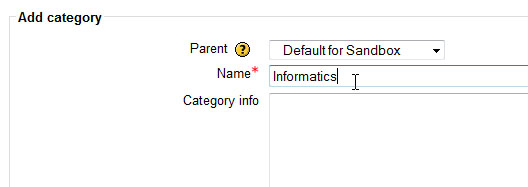Once we have created our categories it is time to add some questions. From the Questions tab, select a category from the Category list which we want to add a question to then select the question type from the Create new question drop-down menu.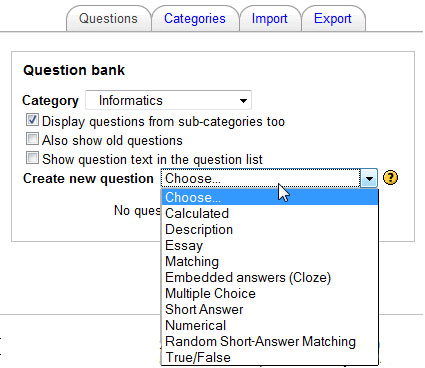Students can choose the answer from a list. Setting options are the followings:## True - False

Only two possible answers: True or False. Write the response into the answer field then set whether it is true or false. By choosing the correct answer maximum points are given to the student while the wrong answer is worth 0 points.Short-answer questions require the student to type an answer -- a world or a phrase. We can choose whether capitalization is important or not. Fill in one answer at least and give each answer a percentage of the grade. Negative points are not acceptable.## NumericalMatching

Multiple questions and matching answers should be given. Fill in at least three questions and answers. When solving the test all possible matching answers can be selected from a drop-down menu.Calculated question

Create a question with two placeholders and a multiplication sign. Instead of numbers use letters in the placeholders.

For example: {x}*kB how many bit?

Enter the formula for the answer using the same placeholders

{x}*8192

Moodle will randomly select values for x therefore the question and the correct answer will be different always.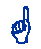Quiz questions and categories can be edited by selecting Questions from the Administration block.

## Adding questions to a quiz

Once we have created our questions, we'll need to add them to the quiz.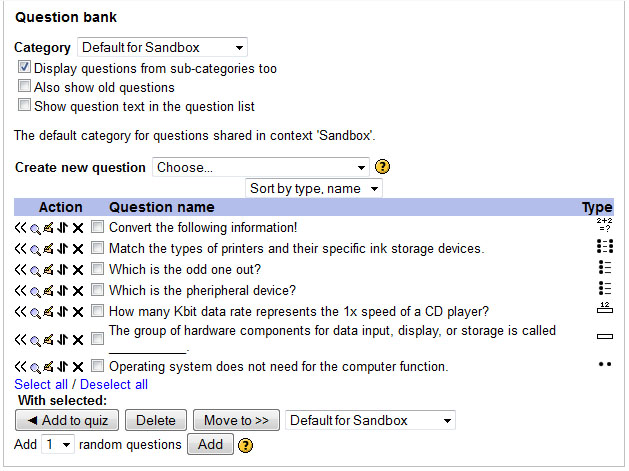Questions we can use can be found on the right side of the screen. We have to move questions we want to add to the quiz to the left side. The chevrons (<< icons) to the left of the questions are used to add individual questions to the quiz. We can also select several questions using the check-boxes on the left of the question list and then click the Add to quiz button at the bottom of the list to add them all at once. If we have a lot of questions we can create a variety of quizzes by clicking on Add random questions button.The same question can be added to a quiz only once but we can also choose questions from other categories (if they have been published when creating the category).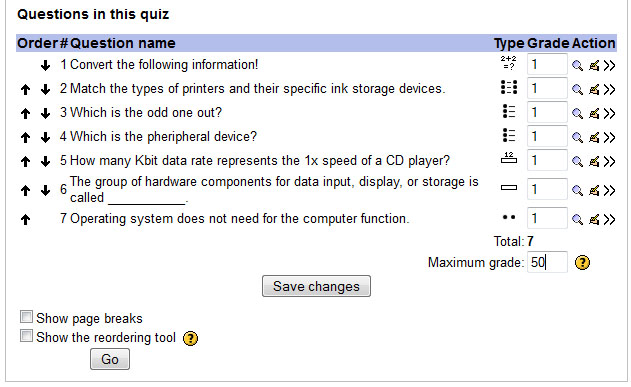After adding questions to a quiz we can change the number of points they carry by setting the relative weight of each question. The maximum grade does not have to be equal to the sum of the grades for the individual questions. The maximum grade for the whole quiz should be set. The system will rescale the result of the quiz from the sum of the grades, the grades achieved by the student and the maximum grade.